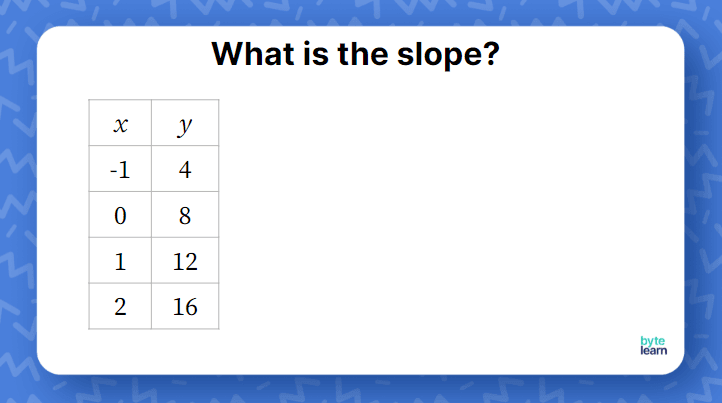ResourcesTestimonials
Plans
ResourcesTestimonials
PlansLesson plan

# Finding the Slope of a Line From a Table Lesson Plan

## Overview

In this lesson, students will learn how to find the slope of a line from a table. Using what they already know about slope, students will examine the patterns found in tables and relate the ordered pairs in the tables to the slope formula. You can expect this lesson to take one `45`-minute class period.

Linear Relationships And Functions
8.F.B.4

## Objective

Students will be able to find the slope of a line from a table.

## Materials

• Teacher slideshow
• Problem Hunt Activity
• Online Practice

## How to Teach Finding the Slope of a Line From a Table

### Warm-up

Activate students’ prior knowledge by asking what they already know about slope from the teacher slideshow. Because it is open-ended, students can share any information they know about slope, whether it is a formula, image, or definition. Help students make connections between what is discussed to help further their understanding.Ask students to share their thoughts with their table partners. As you circulate, you might decide whether students need more practice finding the slope from two points.

### Introduce slope from a table

In the first example, we deliberately introduce a problem to find the slope from a table, where `x`-values in the table go up by `1`.Some students might find the slope without calculating it. They will notice the pattern that the `x`-values go up by `1` and the `y`-values go up by `4`. Some other students might actually apply the slope formula since they just talked about it as part of the warm-up.

### Slope from a table using formula

In the next problem, the `x`-values do not increase at a steady rate, and it is difficult to find the slope by following a pattern.

Some students might want to write down all the consecutive values of `x` and use that to find the slope. Acknowledge that this is a valid way to find the slope. When students who use the slope formula share their thinking, you will want to have a conversation about what is a more efficient method.

### Can we pick any two points?

As students share how they found the slope for the second problem, some will notice that not everybody used the same two points. If nobody brings it up, you can post the work of two students on the board and ask students to write down what is the same, and what is different. This will lead to a conversation about how it does not matter which two points you pick (it will seem magical to some students!!).

Students can also share their rationale for picking their points. Some strategies might evolve as to how do you pick points to make it easiest for them to find slope - for example, students might want to deal with only positive numbers or only with whole numbers rather than fractions.

### Introducing negative slope

In the next problem, students will end up finding a negative slope. The idea is not to spend too much time on this problem. This problem provides a good setup for the next section.

### Predicting the sign of the slope

Help students build an intuitive sense about slope. Usually, when students see a graph, they are able to recognize the sign of the slope depending on the direction of the line.

Ask students if they could have predicted the slope of these two linear relationships. Quite likely, a few students will point out that the `x`- and the `y`-values are moving in the same direction in the first table, and in the opposite direction in the second table. They already know the slopes based on their earlier work. Encourage students to think about the slope before they get too deep into the calculations.

### Problem Hunt activity

To prepare for the Problem Hunt Activity, print Slides `6-10` of the Teacher Slideshow as individual slides and hang them in your room (on walls, cabinets, etc.).

Students can complete the Problem Hunt Activity to practice finding the slope from a table. With the Problem Hunt, students can start at any question and then jump to the question that has the answer to their current question. This allows the activity to be self-checking because students will not return to their first problem until they have answered all of the problems correctly.

The answer key is on the last slide of the slideshow.

## Finding the Slope of a Line From a Table Practice

After you’ve completed the examples with the whole class, it’s time for some independent practice! ByteLearn lets you access tons of practice problems for finding the slope from a table. Check out the online practice and assign it to your students for classwork and/or homework!Finding the Slope of a Line From a Table Practice
Problem 1 of 4
<p>Find the slope of the line using the table.</p><TableUIv2 data-props='{ "headers": [ { "value": "`x`" }, { "value": "`y`" } ], "borders": { "rows": [ 5 ], "cols": [ 2 ] }, "rows": [ [ { "value": "`-6`" }, { "value": "`12`" } ], [ { "value": "`-3`" }, { "value": "`10`" } ], [ { "value": "`6`" }, { "value": "`4`" } ], [ { "value": "`15`" }, { "value": "`-2`" } ] ], "border_color": "rgba(0, 0, 0, 1)"}'></TableUIv2 ><i><p style="color:#666">Write your answer as an integer or simplified fraction.</i></p>

View this practice